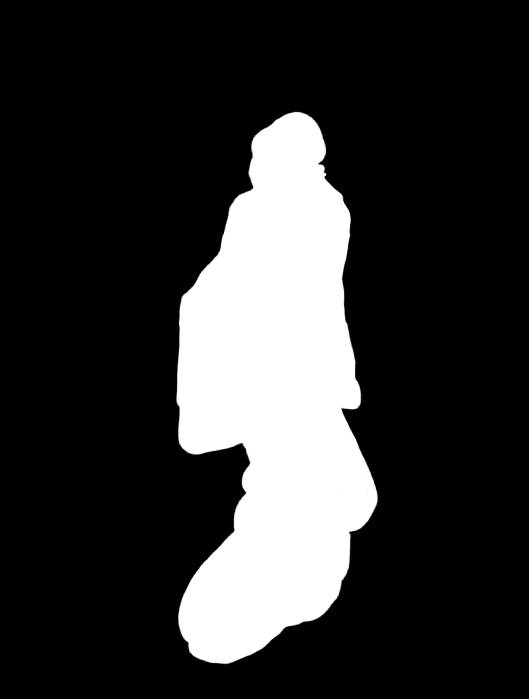## The Problem§

Given an input image $\mathcal{I}$ with dimension $n \times m$, how can we get an output image $\mathcal{I}’$ with size $n \times m’$, where $m’ < m$? Ideally, we’d like $\mathcal{I}’$ to be a good representative of the original image $\mathcal{I}$. However, there is no definitive measure to how good of a representative an image is.

### Content-Oblivious Retargeting§

With scaling, we may stretch or compress an image into a viewport; but this does not preserve the proportions of perceptually “important” content.

With cropping, we reduce the viewport to just the “important” part, but if we desire to keep two objects in the scene that are farther apart than the cropping window, we cannot preserve both.

## Seam Carving§

Then it becomes apparent that we need a retargeting scheme that is “content-aware”, or a method that preserves the important parts of an image.

In seam carving, we remove the unimportant pixels from an image – again, this raises the question of what is an “unimportant” pixel?

One measure is “energy”, which can be expressed as

$$E(\mathcal{I}) = \left|\frac{\partial}{\partial x}\mathcal{I}\right| + \left|\frac{\partial}{\partial y}\mathcal{I}\right|.$$

The human vision system is more sensitive to edges, so by using the gradients as an energy metric should preserve strong contours that are important in human perception.

### Pixel Removal Methods§

todo: include images to better illustrate the drawbacks

1. In optimal carving, we remove all of the lowest energy pixels from the image, leaving only the higher energy pixels – as we remove pixels without preserving context, the image becomes distorted beyond recognition.
2. To reduce an image from $n \times m$ to $n \times m’$ we could remove $m - m’$ pixels from each row – this approach actually gives us an image of $n \times m’$ unlike optimal, but once again the context is ignored giving a bad overall result.
3. Another method is removing $m - m’$ columns from the image, this will also give us an image with dimension $n \times m’$ – this approach gives us an image that is more perceptually consistent, but edges that are not strictly vertical or horizontal do not always line up.
4. Finally, we can choose to find a “seam” along an image, by choosing the lowest energy pixel on the top row, then choosing the lowest energy downward neighbour until we reach the bottom – for most cases, this approach gives us the best results.

### The Seam§

A (vertical) seam is defined to be a connected path of pixels from the top to the bottom of an image, with exactly one pixel per row.

\begin{align*} s^x &= \left\{s_i^x\right\}^n_{i=1} = \left\{(x(i), i)\right\}^n_{i=1}, \forall i, |x(i) - x(i - 1)| \leq 1\\ s^y &= \left\{s_j^y\right\}^n_{j=1} = \left\{(j, y(j))\right\}^n_{j=1}, \forall i, |y(j) - y(ij- 1)| \leq 1 \end{align*}

#### Optimal Seam§

The optimal seam is defined as

$$s^{*} = \argmin_{s} E(s).$$

Unfortunately, this requires us to find all seams in an image, then find the seam with minimal energy – this is extremely inefficient.

However, by using a recursive formulation for a seam, we can use dyanmic programming to get $\mathcal{O}(snm)$ ($s = 3$ in this case).

$$M(i, j) = E(i, j) + \min(M(i - 1, j - 1), M(i - 1, j), M(i - 1, j + 1)).$$

todo: include illustration of dynamic programming approach

### Energy Computation§

There is much freedom in the method of computing the gradients $\frac{\partial}{\partial x}I$ and $\frac{\partial}{\partial y}I$, below are the results of a selection of various finite difference convolution filters.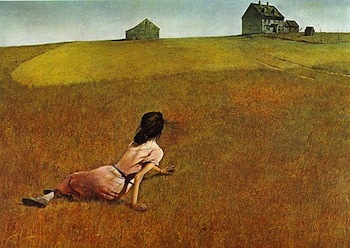OriginalPrewittSobel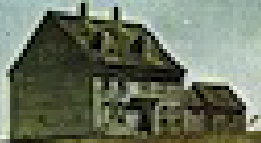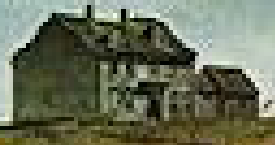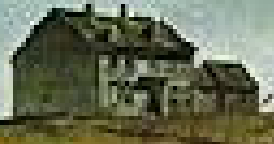RobertsLaplacianRiesz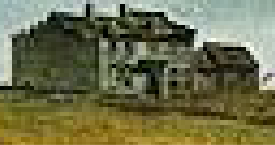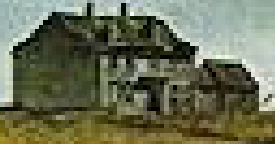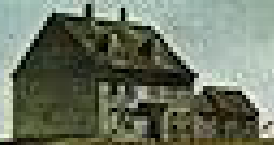For the given input image, the Riesz transform had the least distortion on this building in the background of the image.The Riesz filter is based off of the rotation-invariant Riesz transform and was described in “Riesz Pyramids for Fast Phase-Based Video Magnification” by Wadhwa et al. Then, the gradients using the Riesz filter can be defined by $\mathcal{I}_x = \mathcal{I} \star [-0.5, 0, 0.5]$ and $\mathcal{I}_y = \mathcal{I} \star [-0.5, 0, 0.5]^T$.

## Image Synthesis§

We can also choose to duplicate seams instead of deleting them in order to perform content-aware image expansion.

In order to expand an image by $k$ pixels, we must first perform seam carving to find the first $k$ seams. Then, we can duplicate the $k$ seams to add $k$ pixels to an image dimension. We cannot just find a seam, duplicate it, then repeat by finding a seam on the expanded image – this will most likely result in us picking the same seam many times, this produces an undesirable “streaking” effect instead of content-aware expansion.

The process is described in $\S4.3$ from Avidan and Shamir’s “Seam Carving for Content-Aware Image Resizing”.

Using a print by Utagawa Hiroshige, I was able to extend the width of the image by about 37%; by using a mask, I was able to expand the image without warping the subject of the print.